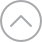# Supported Operators for Various Data Types

The following table contains the list of operators available for different fields:

 Fields Supported Operators Single Line Textbox (Text) \$regex = Contains Multi Line Textbox (Text) \$regex = Contains Rich Text Editor (Text) Single:\$eq = Matches\$ne = Does not matchMultiple:\$eq = Matches\$ne = Does not match\$regex = Contains Markdown (Text) Single:\$eq = Matches\$ne = Does not matchMultiple:\$eq = Matches\$ne = Does not match\$regex = Contains Date (Number) Single:\$eq = Matches\$ne = Does not match\$gt = Greater than\$gte = Greater than or equal to\$lt = Less than\$lte = Less than or equal toMultiple:\$eq = Matches\$ne = Does not match Select (Boolean) Single:\$eq = Matches\$ne = Does not matchMultiple:\$eq = Matches\$ne = Does not match Modular Blocks Not applicable Group Not applicable Link Same as Single Line Textbox Boolean Single:\$eq = Is\$ne = Is notMultiple:\$eq = Is\$ne = Is not Number Single:\$eq = Equals\$ne = Does not equal\$gt = Greater than\$gte = Greater than or equal to\$lt = Less than\$lte = Less than or equal toMultiple:\$eq = Equals\$ne = Does not equal File Single:\$eq = Is\$ne = Is notMultiple:\$eq = Is\$ne = Is not Reference \$in = Is\$nin = Is not Environment tags \$in = Is\$nin = Is not Users \$eq = Is\$ne = Is not Locale \$eq = Is

Note: The Matches operator is case-sensitive.

Thanks for your feedback